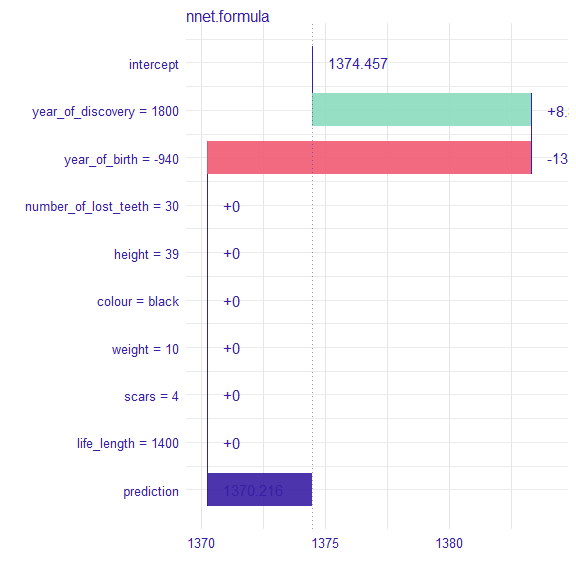# iBreakDown plots for regression models

#### 2019-08-26

Here we will use the dragons data from DALEX package to present the iBreakDown for regression models.

# devtools::install_github("pbiecek/DALEX")
library(DALEX)
library(iBreakDown)

head(dragons)
#>   year_of_birth   height   weight scars colour year_of_discovery
#> 1         -1291 59.40365 15.32391     7    red              1700
#> 2          1589 46.21374 11.80819     5    red              1700
#> 3          1528 49.17233 13.34482     6    red              1700
#> 4          1645 48.29177 13.27427     5  green              1700
#> 5            -8 49.99679 13.08757     1    red              1700
#> 6           915 45.40876 11.48717     2    red              1700
#>   number_of_lost_teeth life_length
#> 1                   25   1368.4331
#> 2                   28   1377.0474
#> 3                   38   1603.9632
#> 4                   33   1434.4222
#> 5                   18    985.4905
#> 6                   20    969.5682
new_observation <- dragons_test[1,]
new_observation
#>   year_of_birth   height   weight scars colour year_of_discovery
#> 1          -938 39.18619 10.02391     4  black              1800
#>   number_of_lost_teeth life_length
#> 1                   30     1375.38

## Linear regression

First, we fit a model.

m_lm <- lm(life_length ~ . , data = dragons)

To understand the factors that drive predictions for a single observation we use the iBreakDown package.

Now, we create an object of the break_down class. If we want to plot distributions of partial predictions, set keep_distributions = TRUE.

bd_lm <- local_attributions(m_lm,
data = dragons_test,
new_observation =  new_observation,
keep_distributions = TRUE)

We can simply print the result.

bd_lm
#>                               contribution
#> lm: intercept                     1356.562
#> lm: scars = 4                     -235.221
#> lm: number_of_lost_teeth = 30      205.037
#> lm: year_of_birth = -940            22.193
#> lm: height = 39                     11.296
#> lm: colour = black                  10.856
#> lm: weight = 10                     -9.217
#> lm: year_of_discovery = 1800         4.668
#> lm: life_length = 1400               0.000
#> lm: prediction                    1366.174

Or plot the result which is more clear.

plot(bd_lm)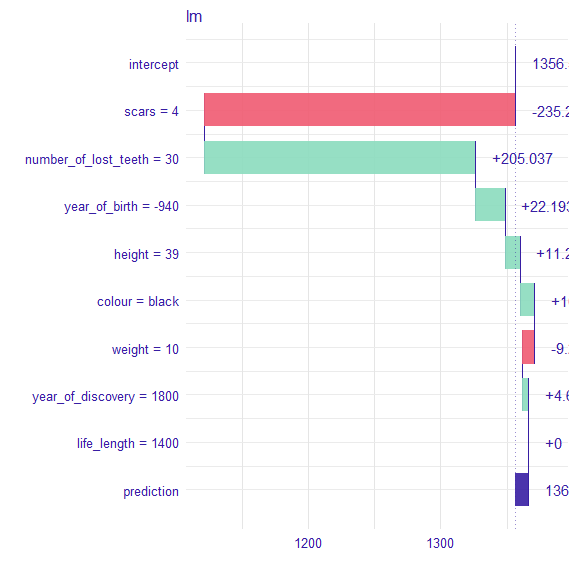Use the baseline parameter to set the origin of plots.

plot(bd_lm, baseline = 0)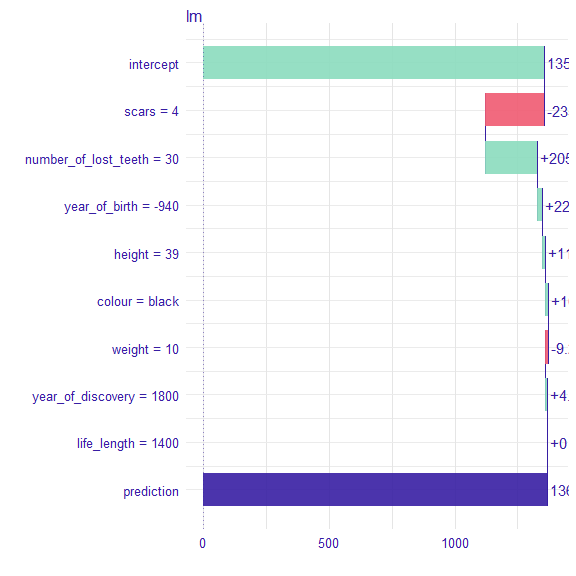Use the plot_distributions parameter to see distributions of partial predictions.

plot(bd_lm, plot_distributions = TRUE)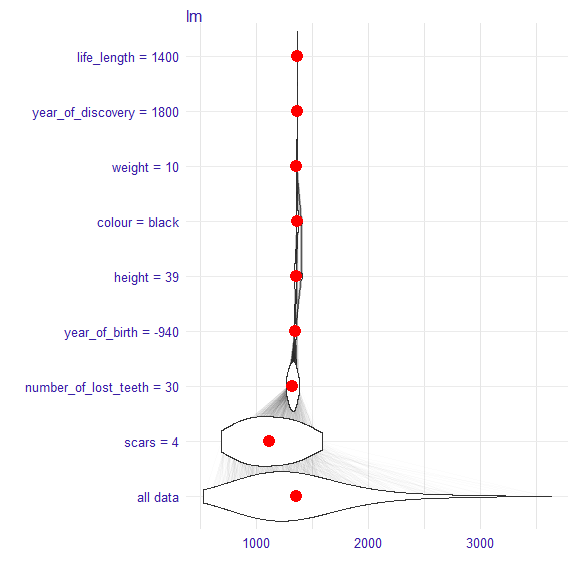For another types of models we proceed analogously. However, sometimes we need to create custom predict function (see nnet example).

## randomForest

library(randomForest)

m_rf <- lm(life_length ~ . , data = dragons)

data = dragons_test,
new_observation =  new_observation)

head(bd_rf)
#>                               contribution
#> lm: intercept                     1356.562
#> lm: scars = 4                     -235.221
#> lm: number_of_lost_teeth = 30      205.037
#> lm: year_of_birth = -940            22.193
#> lm: height = 39                     11.296
#> lm: colour = black                  10.856
plot(bd_rf)## SVM

library(e1071)

m_svm <- svm(life_length ~ . , data = dragons)

data = dragons_test,
new_observation =  new_observation)

plot(bd_svm)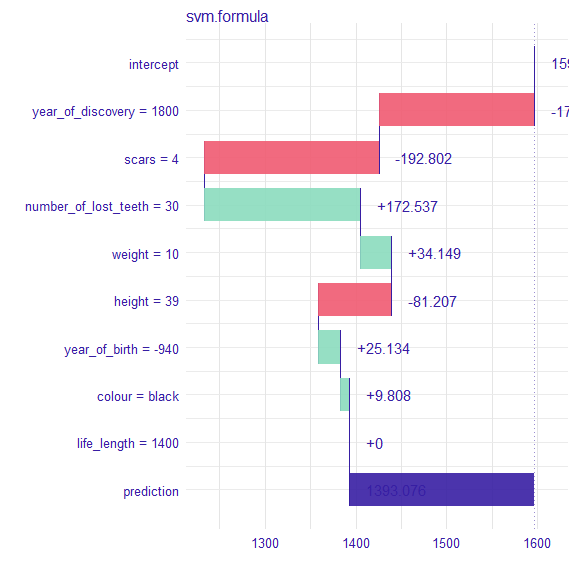## knn

library(caret)

m_knn <- knnreg(life_length ~ . , data = dragons)

data = dragons_test,
new_observation =  new_observation)

plot(bd_knn)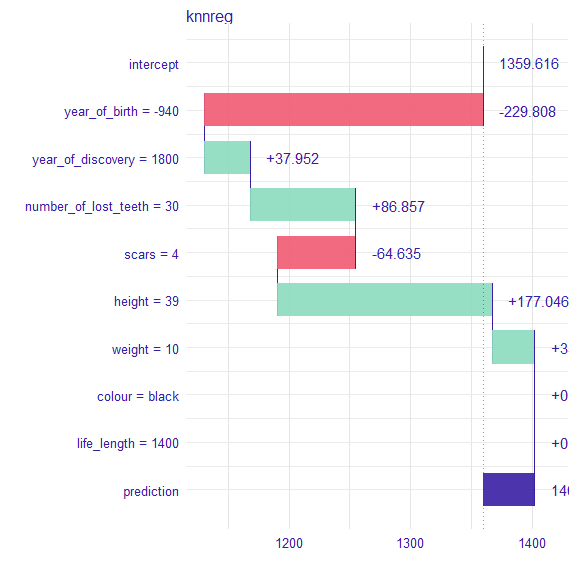## nnet

When you use nnet package for regression, remember to normalize the resposne variable, in such a way that it is from interval $$(0,1)$$.

In this case, creating custom predict function is also needed.

library(nnet)

x <- max(abs(dragons\$life_length))
digits <- floor(log10(x))
normalizing_factor <- round(x, -digits)
m_nnet <- nnet(life_length/normalizing_factor ~ . , data = dragons, size = 10, linout = TRUE)
#> # weights:  111
#> initial  value 11078.237155
#> iter  10 value 27.594748
#> final  value 27.461013
#> converged
p_fun <- function(model, new_observation){
predict(model, newdata = new_observation)*normalizing_factor
}

plot(bd_nnet)